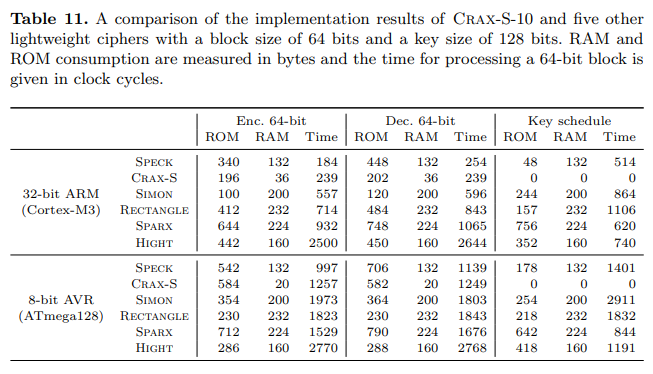# CRAX, a Classic Block Cipher

Crax-S-10 is a 64-bit block cipher that uses a 128-bit secret key intended for the most constrained micro-controllers. Since its key schedule is very simple and does not have to be precomputed, it is one of the fastest 64-bit lightweight block ciphers in software, beaten only for messages longer than 72 bytes by the NSA cipher Speck. Due to this simple key schedule, it consumes lower RAM than Speck. While the family of tweakable block ciphers Skinny can be considered as an academic alternative to the NSA cipher Simon in terms of hardware efficiency, Crax-S-10 can be seen as an academic alternative to Speck in terms of software efficiency.

## Description

Crax-S-10 is built as a key-alternating cipher on top of the Alzette ARX-box. It has 10 steps (calls to Alzette with 5 different constants) alternated with key and constant XORs.

The following C code is a reference implementation for Crax-S-10.

#define ROT(x, n) (((x) >> (n)) | ((x) << (32-(n))))

#define ALZETTE(x, y, c)                    \
(x) += ROT((y), 31), (y) ^= ROT((x), 24), \
(x) ^= (c),                               \
(x) += ROT((y), 17), (y) ^= ROT((x), 17), \
(x) ^= (c),                               \
(x) += (y),          (y) ^= ROT((x), 31), \
(x) ^= (c),                               \
(x) += ROT((y), 24), (y) ^= ROT((x), 16), \
(x) ^= (c)

#define ALZETTE_INV(x, y, c)                \
(x) ^= (c),                               \
(y) ^= ROT((x), 16), (x) -= ROT((y), 24), \
(x) ^= (c),                               \
(y) ^= ROT((x), 31), (x) -= (y),          \
(x) ^= (c),                               \
(y) ^= ROT((x), 17), (x) -= ROT((y), 17), \
(x) ^= (c),                               \
(y) ^= ROT((x), 24), (x) -= ROT((y), 31)

#define N_STEPS 10

static const uint32_t RCON = {                             \
0xB7E15162, 0xBF715880, 0x38B4DA56, 0x324E7738, 0xBB1185EB };

void craxs10_enc_ref(uint32_t *xword, uint32_t *yword, const uint32_t *key)
{
int step;

for (step = 0; step < NSTEPS; step++) {
xword ^= step;
if ((step % 2) == 0) {
xword ^= key;
yword ^= key;
} else {
xword ^= key;
yword ^= key;
}
ALZETTE(xword, yword, RCON[step%5]);
}
xword ^= key;
yword ^= key;
}

void craxs10_dec_ref(uint32_t *xword, uint32_t *yword, const uint32_t *key)
{
int step;

xword ^= key;
yword ^= key;
for (step = NSTEPS-1; step >= 0; step--) {
ALZETTE_INV(xword, yword, RCON[step%5]);
if ((step % 2) == 0) {
xword ^= key;
yword ^= key;
} else {
xword ^= key;
yword ^= key;
}
xword ^= step;
}
}


## Performance

Crax-S-10 is a very lightweight block cipher, arguably one of the the lightest ever reported in the literature when it comes to micro-controller implementations. The code size, RAM consumption, and execution time of Crax-S-10 on an 8-bit AVR and a 32-bit ARM Cortex-M3 micro-controller are summarized in Table 11, along with those of five other lightweight block ciphers, namely Speck64/128, Simon-64/128, Rectangle, Sparx, and Hight.